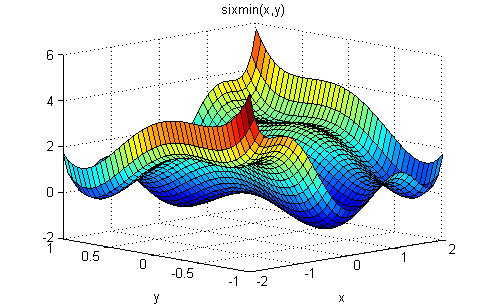## Run the Solver

### Optimize by Calling run

Running a solver is nearly identical for `GlobalSearch` and `MultiStart`. The only difference in syntax is `MultiStart` takes an additional input describing the start points.

For example, suppose you want to find several local minima of the `sixmin` function

sixmin = 4x2 – 2.1x4 + x6/3 + xy – 4y2 + 4y4.This function is also called the six-hump camel back function . All the local minima lie in the region –3 ≤ x,y ≤ 3.

### Example of Run with GlobalSearch

To find several local minima of the `sixmin` function using `GlobalSearch`, enter:

```% % Set the random stream to get exactly the same output % rng(14,'twister') gs = GlobalSearch; opts = optimoptions(@fmincon,'Algorithm','interior-point'); sixmin = @(x)(4*x(1)^2 - 2.1*x(1)^4 + x(1)^6/3 ... + x(1)*x(2) - 4*x(2)^2 + 4*x(2)^4); problem = createOptimProblem('fmincon','x0',[-1,2],... 'objective',sixmin,'lb',[-3,-3],'ub',[3,3],... 'options',opts); [xming,fming,flagg,outptg,manyminsg] = run(gs,problem);```

The output of the run (which varies, based on the random seed):

```xming,fming,flagg,outptg,manyminsg xming = 0.0898 -0.7127 fming = -1.0316 flagg = 1 outptg = struct with fields: funcCount: 2115 localSolverTotal: 3 localSolverSuccess: 3 localSolverIncomplete: 0 localSolverNoSolution: 0 message: 'GlobalSearch stopped because it analyzed all the trial po...' manyminsg = 1x2 GlobalOptimSolution array with properties: X Fval Exitflag Output X0```

### Example of Run with MultiStart

To find several local minima of the `sixmin` function using 50 runs of `fmincon` with `MultiStart`, enter:

```% % Set the random stream to get exactly the same output % rng(14,'twister') ms = MultiStart; opts = optimoptions(@fmincon,'Algorithm','interior-point'); sixmin = @(x)(4*x(1)^2 - 2.1*x(1)^4 + x(1)^6/3 ... + x(1)*x(2) - 4*x(2)^2 + 4*x(2)^4); problem = createOptimProblem('fmincon','x0',[-1,2],... 'objective',sixmin,'lb',[-3,-3],'ub',[3,3],... 'options',opts); [xminm,fminm,flagm,outptm,manyminsm] = run(ms,problem,50);```

The output of the run (which varies based on the random seed):

```xminm,fminm,flagm,outptm,manyminsm xminm = 0.0898 -0.7127 fminm = -1.0316 flagm = 1 outptm = struct with fields: funcCount: 2034 localSolverTotal: 50 localSolverSuccess: 50 localSolverIncomplete: 0 localSolverNoSolution: 0 message: 'MultiStart completed the runs from all start points.…' manyminsm = 1x6 GlobalOptimSolution array with properties: X Fval Exitflag Output X0```

In this case, `MultiStart` located all six local minima, while `GlobalSearch` located two. For pictures of the `MultiStart` solutions, see Visualize the Basins of Attraction.

Watch now